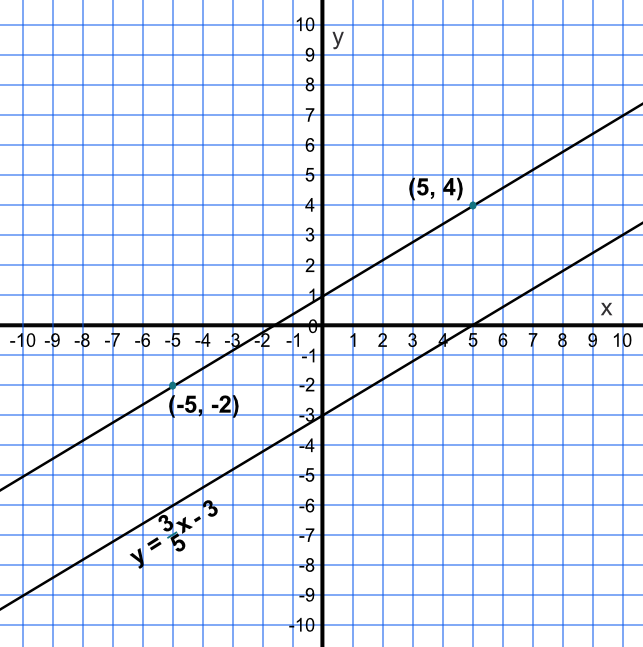Parallel Lines

## Parallel Lines

Parallel lines have the same gradient, or slope, but different intercept points on the y-axis. If both lines are written in the form y=mx+c, then the value of m, the gradient, will be the same for both lines.

A set of collinear points all exist on the same line.

## Example 1

Is the line defined by two points, (-5,-2) and (5,4), parallel to the line given by the equation y = frac(3)(5)x - 3?

Both gradients are the same, so the lines are parallel.Answer: Yes. The gradient between these points is frac(up)(along) = frac(4 - -2)(5 - -5) = frac(6)(10) = frac(3)(5).

## Example 2

A line is given by an equation y = -2x + 4. Give the equation of a line, parallel to the given equation, that passes through (0, 5).

The gradient is the same in both equations. Substitute the point into the equation:

5 = -2xx0 + c therefore c=5

The equation of the line is y = -2x + 5

Answer: y = -2x + 5